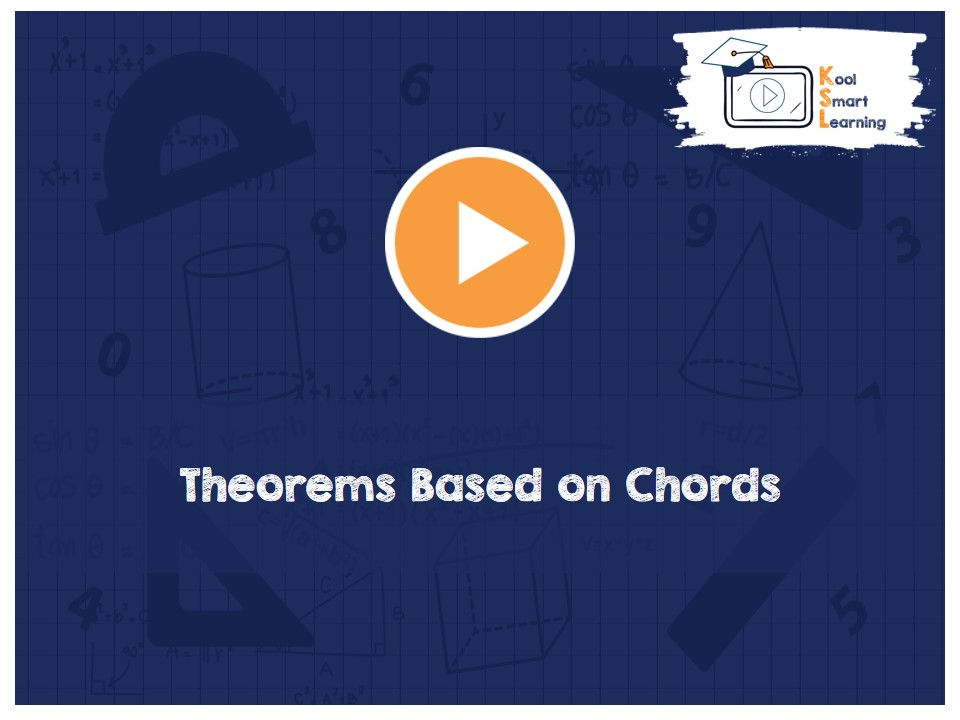At KoolSmartLearning, we intend to harness the power of online education to make learning easy.## Theorems Based on Chords

/  Theorems Based on Chords## Theorems Based on Chords

The video discusses the theorems based on Chords of a Circle along with proof of each of the theorem. The theorems covered are equal chords subtend equal angle at the center and its converse theorem, perpendicular from the center to the chord bisects the chord & its converse theorem, equal chords of a circle are equidistant from the center and its converse theorem.

More Related Videos